COVID-19 UPDATE: Bliley continuing to stay open with full operation. Read Full Statement

## Inside Frequency Control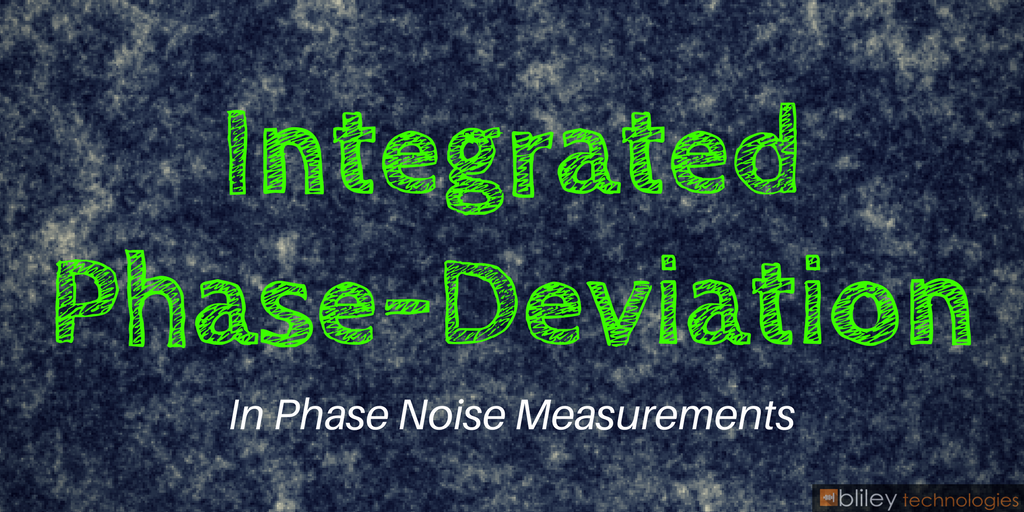With the advances of RF technology over the last many years, most modern spectrum analyzers can automatically detect changes in phase noise levels (mostly of sine wave signals) measured in units of dBc or rads. It’s always a good idea for RF engineers to have a better understanding of how modern spectrum analyzers measure such deviations in phase noise in the first place. Or better yet, understand how to optimize a spectrum analyzer’s measurements even further. (Don’t let those spectrum analyzers outsmart that great brain of yours)! Believe it or not, many spectrum analyzer readings of phase noise can actually be significantly off.

Measuring phase noise deviations is actually easier than it may first sound. All you really have to do is learn how to calculate what’s called the Root Mean Square (RMS). This is done by calculating the ratio of power from the Single Sideband (SSB) phase noise to the carrier. I recommend checking outbefore continuing if you feel unfamiliar with phase noise.

Angle Modulating and The Bessel Function

First, let’s jump back a little and discuss the relationship of the modulating index of an angle modulated signal to the curves of the Bessel function. This will help determine the Root Mean Square later on.

Angle Modulating

Angle-modulated signals are signals with phase and frequency modulation in a carrier and are identified by a modulation index (m). The easiest way to determine this modulation index is by finding the peak phase deviation.

Peak phase deviation = The ratio of the peak frequency deviation to the modulating frequency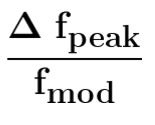The Bessel Function

An angle modulating sine wave signal of a given carrier frequency, also at a given modulating frequency, will create an endless number of sidebands. The amplitudes of the modulated carrier and sidebands can be found by looking at the modulation index discussed above as well as the curves of the Bessel function J0 - Jn (As seen below).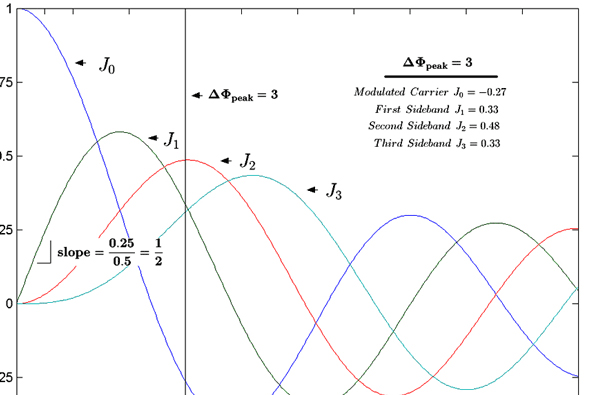Source: mwrf.com

The chart above shows the Bessel function curves (J0-J3). In this example, the black vertical line is at a modulation index of 3 (same as 3 rads). With the intersection of the individual Bessel curves through the mod index, it is easy to see the relative amplitude of the modulated carrier as well as the sidebands to the unmodulated carrier. It’s important to note this is on a voltage scale.

So, for this example, the relative amplitudes for each individual curve where they cross the mod index (set at 3) are:

• J0 (Modulated carrier) = -0.26
• J1 (1st Sideband) = 0.33
• J2 (2nd Sideband) = 0.48
• J3 (3rd Sideband) = ~0.33

Through a Spectrum Analyzer

Unfortunately, most spectrum analyzers show the phase noise results in the RMS phase deviation without the peak phase deviation.

Here’s a nifty little formula you can use. It converts the peak deviation to RMS: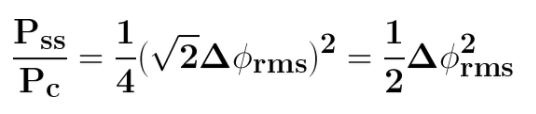Pss = Power of Single Sideband

Pc = Power of carrier

Lastly, the following equation determines the carrier’s RMS phase deviation when modulated with a sine wave signal (measured on a relative power scale):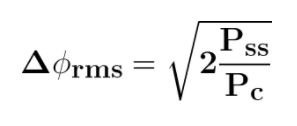How to Calculate the Integrated Phase Deviation from Phase Noise

This may also be a good time to check out our Ultimate Guide to Phase Noise if you haven’t already before continuing.

To calculate the integrated phase deviation, we must introduce the noise density of the power in the SSB phase noise of a given signal. The Integrated Bandwidth Method will help determine this phase noise.

First, select a range of offset (Interval between fstart to fstop). The power is then integrated between the 2 frequency points. Do this by using the following formula: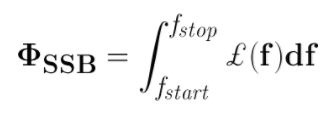£ (f) = Noise density of power in SSB phase noise (Power-density ratio units)

We want to calculate the power of the single-sideband, so use: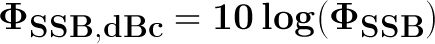Use this formula to calculate the entire frequency band (2X as large as single-sideband)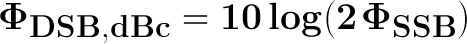You may notice that the Pss/Pc is the same power as the single-sideband ratio, So: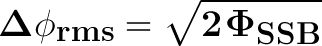This calculation is actually used in spectrum analyzers to show the integrated phase-noise measurements (in radians).

Phase noise test sets can be quite expensive compared to spectrum analyzers, but if purchased, can allow your phase noise readings to be much more accurate. And of course, would help save time doing all the calculations.

Looking for low phase nosie, even in high vibration environments? Our Poseidon 2 OCXO may be the perfect choice for your application.Topics: RF Technology